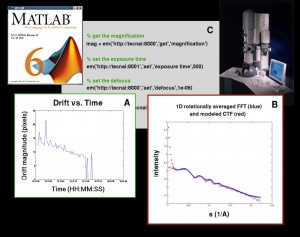# A Matlab Interface to the Tecnai TEMA Matlab Interface to the Tecnai TEM

We have developed a Matlab interface, emMatlab, to control and acquire images from a Tecnai TEM connected to either a Tietz or a Gatan CCD camera. The following plots and sample code illustrate how emMatlab can be implemented. (A) This plot measures drift as a function of time. The system was perturbed by briefly adding a heat load to the anti-contaminator blades of the Tecnai TEM. (B) A 1D plot of the filtered and rotationally averaged FFT of an acquired image (blue). A plot of a coarsely fit CTF (red) to the raw data (blue). (C) Sample Matlab interface code that controls and acquires images from a Tecnai TEM connected to either a Tietz or Gatan CCD camera.

Images courtesy of NRAMM, Mathworks, and FEI.